Anúncio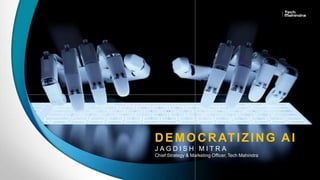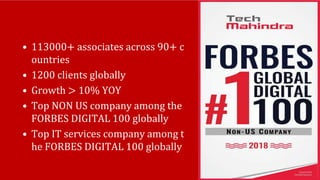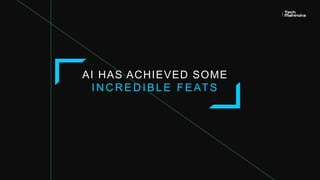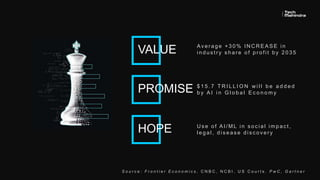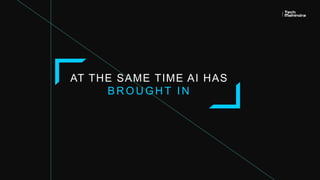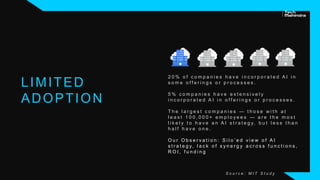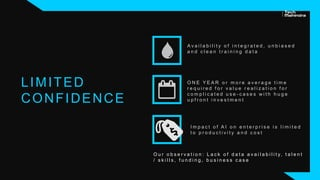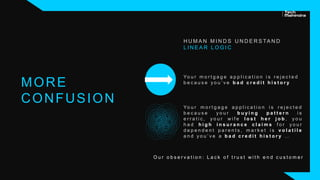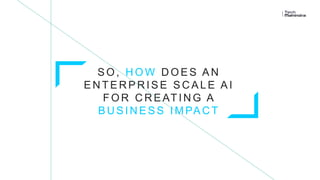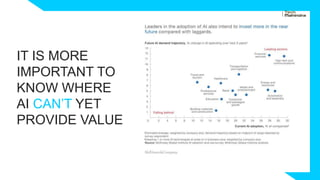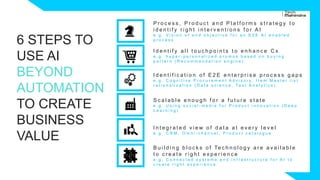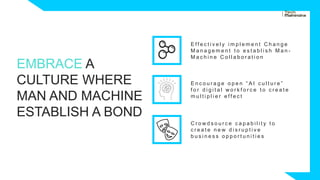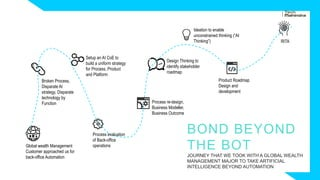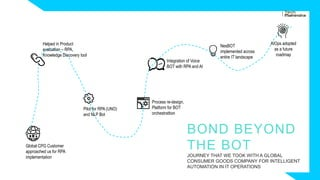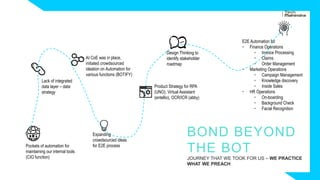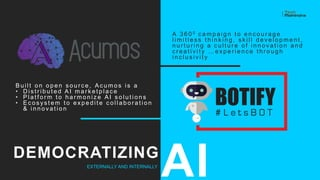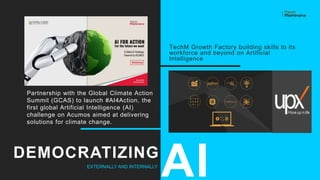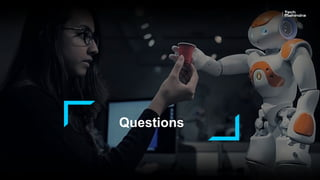1 de 18
Anúncio

### Keynote - Jagdish Mitra - Democratizing AI - H2O AI World London 2018

1. DEMOCRATIZING AI J A G D I S H M I T R A Chief Strategy & Marketing Officer, Tech Mahindra
2. • 113000+ associates across 90+ c ountries • 1200 clients globally • Growth > 10% YOY • Top NON US company among the FORBES DIGITAL 100 globally • Top IT services company among t he FORBES DIGITAL 100 globally
3. Copyright © 2018 | Tech Mahindra | All rights reserved. AI HAS ACHIEVED SOME I N C R E D I B L E F E AT S
4. Copyright © 2018 | Tech Mahindra | All rights reserved. Av e r a g e + 3 0 % I N C R E A S E i n i n d u s t r y s h a r e o f p r o f i t b y 2 0 3 5 \$ 1 5 . 7 T R I L L I O N w i l l b e a d d e d b y A I i n G l o b a l E c o n o m yPROMISE VALUE HOPE S o u r c e : F r o n t i e r E c o n o m i c s , C N B C , N C B I , U S C o u r t s . P w C , G a r t n e r U s e o f A I / M L i n s o c i a l i m p a c t , l e g a l , d i s e a s e d i s c o v e r y
6. LIMITED ADOPTION 2 0 % o f c o m p a n i e s h a v e i n c o r p o r a t e d A I i n s o m e o f f e r i n g s o r p r o c e s s e s . 5 % c o m p a n i e s h a v e e x t e n s i v e l y i n c o r p o r a t e d A I i n o f f e r i n g s o r p r o c e s s e s . T h e l a r g e s t c o m p a n i e s — t h o s e w i t h a t l e a s t 1 0 0 , 0 0 0 + e m p l o y e e s — a r e t h e m o s t l i k e l y t o h a v e a n A I s t r a t e g y, b u t l e s s t h a n h a l f h a v e o n e . O u r O b s e r v a t i o n : S i l o ’ e d v i e w o f A I s t r a t e g y, l a c k o f s y n e r g y a c r o s s f u n c t i o n s , R O I , f u n d i n g S o u r c e : M I T S t u d y
7. LIMITED CONFIDENCE I m p a c t o f A I o n e n t e r p r i s e i s l i m i t e d t o p r o d u c t i v i t y a n d c o s t O N E Y E A R o r m o r e a v e r a g e t i m e r e q u i r e d f o r v a l u e r e a l i z a t i o n f o r c o m p l i c a t e d u s e - c a s e s w i t h h u g e u p f r o n t i n v e s t m e n t A v a i l a b i l i t y o f i n t e g r a t e d , u n b i a s e d a n d c l e a n t r a i n i n g d a t a O u r o b s e r v a t i o n : L a c k o f d a t a a v a i l a b i l i t y, t a l e n t / s k i l l s , f u n d i n g , b u s i n e s s c a s e
8. H U M A N M I N D S U N D E R S TA N D L I N E A R L O G I C Yo u r m o r t g a g e a p p l i c a t i o n i s r e j e c t e d b e c a u s e y o u r b u y i n g p a t t e r n i s e r r a t i c , y o u r w i f e l o s t h e r j o b , y o u h a d h i g h i n s u r a n c e c l a i m s f o r y o u r d e p e n d e n t p a r e n t s , m a r k e t i s v o l a t i l e a n d y o u ’ v e a b a d c r e d i t h i s t o r y … Yo u r m o r t g a g e a p p l i c a t i o n i s r e j e c t e d b e c a u s e y o u ’ v e b a d c r e d i t h i s t o r y MORE CONFUSION O u r o b s e r v a t i o n : L a c k o f t r u s t w i t h e n d c u s t o m e r
9. Copyright © 2018 | Tech Mahindra | All rights reserved. S O , H O W D O E S A N E N T E R P R I S E S C A L E A I F O R C R E AT I N G A B U S I N E S S I M PA C T
10. IT IS MORE IMPORTANT TO KNOW WHERE AI CAN’T YET PROVIDE VALUE
11. 6 STEPS TO USE AI BEYOND AUTOMATION TO CREATE BUSINESS VALUE I d e n t i f y a l l t o u c h p o i n t s t o e n h a n c e C x e . g . h y p e r - p e r s o n a l i z e d p r o m o s b a s e d o n b u y i n g p a t t e r n ( R e c o m m e n d a t i o n e n g i n e ) P r o c e s s , P r o d u c t a n d P l a t f o r m s s t r a t e g y t o i d e n t i f y r i g h t i n t e r v e n t i o n s f o r A I e . g . V i s i o n o f e n d o b j e c t i v e f o r a n E 2 E A I e n a b l e d p r o c e s s S c a l a b l e e n o u g h f o r a f u t u r e s t a t e e . g . U s i n g s o c i a l - m e d i a f o r P r o d u c t i n n o v a t i o n ( D e e p L e a r n i n g ) I d e n t i f i c a t i o n o f E 2 E e n t e r p r i s e p r o c e s s g a p s e . g . C o g n i t i v e P r o c u r e m e n t A d v i s o r y , I t e m M a s t e r l i s t r a t i o n a l i z a t i o n ( D a t a s c i e n c e , Te x t A n a l y t i c s ) I n t e g r a t e d v i e w o f d a t a a t e v e r y l e v e l e . g . C R M , O m n i - c h a n n e l , P r o d u c t c a t a l o g u e B u i l d i n g b l o c k s o f Te c h n o l o g y a r e a v a i l a b l e t o c r e a t e r i g h t e x p e r i e n c e e . g . C o n n e c t e d s y s t e m s a n d i n f r a s t r u c t u r e f o r A I t o c r e a t e r i g h t e x p e r i e n c e
12. EMBRACE A CULTURE WHERE MAN AND MACHINE ESTABLISH A BOND E n c o u r a g e o p e n “ A I c u l t u r e ” f o r d i g i t a l w o r k f o r c e t o c r e a t e m u l t i p l i e r e f f e c t E f f e c t i v e l y i m p l e m e n t C h a n g e M a n a g e m e n t t o e s t a b l i s h M a n - M a c h i n e C o l l a b o r a t i o n C r o w d s o u r c e c a p a b i l i t y t o c r e a t e n e w d i s r u p t i v e b u s i n e s s o p p o r t u n i t i e s
13. Global wealth Management Customer approached us for back-office Automation Broken Process, Disparate AI strategy, Disparate technology by Function Setup an AI CoE to build a uniform strategy for Process, Product and Platform Ideation to enable unconstrained thinking (“AI Thinking”) Process evaluation of Back-office operations Process re-design, Business Modeller, Business Outcome Design Thinking to identify stakeholder roadmap Product Roadmap Design and development RITA BOND BEYOND THE BOT JOURNEY THAT WE TOOK WITH A GLOBAL WEALTH MANAGEMENT MAJOR TO TAKE ARTIFICIAL INTELLIGENCE BEYOND AUTOMATION
14. Global CPG Customer approached us for RPA implementation Helped in Product evaluation – RPA, Knowledge Discovery tool NesBOT implemented across entire IT landscape Pilot for RPA (UNO) and NLP Bot Process re-design, Platform for BOT orchestrattion Integration of Voice BOT with RPA and AI AIOps adopted as a future roadmap BOND BEYOND THE BOT JOURNEY THAT WE TOOK WITH A GLOBAL CONSUMER GOODS COMPANY FOR INTELLIGENT AUTOMATION IN IT OPERATIONS
15. Pockets of automation for maintaining our internal tools (CIO function) Lack of integrated data layer – data strategy AI CoE was in place, initiated crowdsourced ideation on Automation for various functions (BOTIFY) Expanding crowdsourced ideas for E2E process Product Strategy for RPA (UNO), Virtual Assistant (entellio), OCR/ICR (abby) Design Thinking to identify stakeholder roadmap E2E Automation for • Finance Operations • Invoice Processing • Claims • Order Management • Marketing Operations • Campaign Management • Knowledge discovery • Inside Sales • HR Operations • On-boarding • Background Check • Facial Recognition BOND BEYOND THE BOT JOURNEY THAT WE TOOK FOR US – WE PRACTICE WHAT WE PREACH
16. A 3 6 0 0 c a m p a i g n t o e n c o u r a g e l i m i t l e s s t h i n k i n g , s k i l l d e v e l o p m e n t , n u r t u r i n g a c u l t u r e o f i n n o v a t i o n a n d c r e a t i v i t y … e x p e r i e n c e t h r o u g h i n c l u s i v i t y B u i l t o n o p e n s o u r c e , A c u m o s i s a • D i s t r i b u t e d A I m a r k e t p l a c e • P l a t f o r m t o h a r m o n i z e A I s o l u t i o n s • E c o s ys t e m t o e x p e d i t e c o l l a b o r a t i o n & i n n o v a t i o n DEMOCRATIZING EXTERNALLY AND INTERNALLY AI
17. TechM Growth Factory building skills to its workforce and beyond on Artificial Intelligence DEMOCRATIZING EXTERNALLY AND INTERNALLY AI Partnership with the Global Climate Action Summit (GCAS) to launch #AI4Action, the first global Artificial Intelligence (AI) challenge on Acumos aimed at delivering solutions for climate change.
18. Questions

### Notas do Editor

1. COO – example includes CEMEX where we
2. COO – example includes CEMEX where we
3. COO – example includes CEMEX where we
4. COO – example includes CEMEX where we
Anúncio